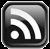# LEARN MS-ACCESS TIPS AND TRICKS

Learn Microsoft Access Advanced Programming Techniques, Tips and Tricks.

### Calculating Time Difference

How do we calculate difference of time in Hours, Minutes and Seconds between two time period? The time period can be on the same date or across dates.

Example-1:

Start Time: 21-03-2012 12:30:45

End Time:   21-03-2012 13:30:15

Example-2:

Start Time: 21-03-2012 12:30:45

End Time: 24-03-2012 15:15:15

In the first example the date values in the Start Time and End Time, looks like it is not important, because both are same, to calculate the time difference between them. We can subtract the Start Time value 12:30:45 from the End Time value 13:30:15 and will get the result 00:59:30.  But, if the end time is 01:30:15 past mid-night then how do we calculate the time difference?

But first, how the time changes to zero after 23:59:59 Hrs at night and how this value is held in computer’s memory.  With this basic knowledge working with the date and time values become very easy.

Everybody knows that a day is equals to 24 hours, 1 hour equals to 60 Minutes, 1 Minute equals to 60 Seconds and further if we go to a smaller denomination 1 second equals to 100 milliseconds. If you would like to find out how February gets 29 days (everybody knows about it) but why every 100th Year (like 1700, 1800,1900 or 2100) is not a Leap Year, how every 400th Year become Leap Year and why every 4000th year is not Leap Year, Click here to find out!

So, keeping aside the milliseconds part 1 Day = 86400 Seconds (24 x 60 x 60).  In other words 1 Second = 1/86400 = 0.0000115740740741 Days. Exactly 1 second after midnight the computer’s internal time value will be 0.0000115740740741 and this value keeps adding to the time value at every second interval.  This value can be divided by 100 to get the value equal to 1 millisecond and we could say that value is added to the time value at every millisecond interval. At 12:00 Noon the internal time value is 0.5 (half a day) and at 23:59:59 the internal time value will be 0.999988425926 (in days).  Again this will reset to 0 at 12:00:00 midnight.

To find out the time difference between time values spread across different dates the date value also should go along with the time value as shown in Example-2 above.  The date-value also mapped in computer’s memory as a continuous number starting 1 from 31-12-1899.  If you create a Date/Time field in your Table having 0 as default value then you will find the date appearing in that field as 30-12-1899, if not filled-in with a date.

The date 21-03-2012 equals to the date number 40989 and the Start Time (Example-2) in memory will be 40989.521354166700, the number after the decimal point is the time value.  Since, the Date and Time values held in memory are real numbers it is easy to find out the difference between them.  All you have to do is to subtract one number from other.  This works with Date/Time Values on the same day or on different dates.

Let us find out the time difference between the date and time values in Example-2:

StartTime = #21-03-2012 12:30:45# = 40989.521354166700

This is the kind of value (Current Date and Time) returned with the function Now().  You can format this value as date alone (dd-mm-yyyy) or time alone (hh:nn:ss) or both combined - format(now(),”dd-mm-yyyy hh:nn:ss”).

Use ? format(StartTime,”0.000000000000”) to display the Date/Time value as a real number from StartTime Variable in the Debug Window.

EndTime = #24-03-2012 15:15:15# = 40992.635590277800

Difference = (EndTime - StartTime) = 3.114236111112 days

So, the result value we get in days, doesn’t matter the date is same or different dates.  If the date is same you will get the result in days something like 0.9999999.  All we have to do is to convert this days into Hours, Minutes and Seconds.

Total_Seconds = Difference x 86400 (difference in days converted into total seconds) = 269070.000000077 Seconds

Hours = Int(Total_Seconds / 3600) = 74 Hrs.

Minutes = int((Total_Second MOD 3600)/60) = 44 Min.

Seconds = Total_Seconds MOD 60 = 30 sec.

If we assemble all these information into the form of a small function we can easily find the time difference in Hours, Minutes and Seconds  by passing the Start & End Date/Time Values to the Function as parameters.

Copy and paste the following Function Code into the Standard Module and save it:

```Public Function HrsMin(ByVal startDate As Date, ByVal endDate As Date) As String
Dim diff As Double
Dim difHrs As Integer
Dim difMin As Integer
Dim difSec As Integer
diff = (endDate - startDate) * 86400
difHrs = Int(diff / 3600)
difMin = Int((diff Mod 3600) / 60)
difSec = (diff Mod 60)
HrsMin = Format(difHrs, "00") & ":" & Format(difMin, "00") & ":" & Format(difSec, "00")
End Function
```

You may call the Function HrsMin() directly from the Debug Window, as shown below, to test the code:

```? HrsMin(#21-03-2012 12:30:45#,#24-03-2012 15:15:15#)
```
Result: 74:44:30

You may call the function from a Textbox in the Form like:

```= HrsMin([StartDate],[EndDate])
```

Or from the Query Column like:

```HMS:HrsMin([StartDate],[EndDate])
```

Or you may run it from VBA Code:

```strHMS = HrsMin(dtSDate,dtEDate)
```
Technorati Tags:
Share:

## TranslateSubscribe in a readerYour email address:

Delivered by FeedBurner

## Featured Post

### Add Class Objects as Dictionary Items

We have learned the fundamentals of Dictionary Object, done trial runs for sorting simple items and displayed Access Table records through D...